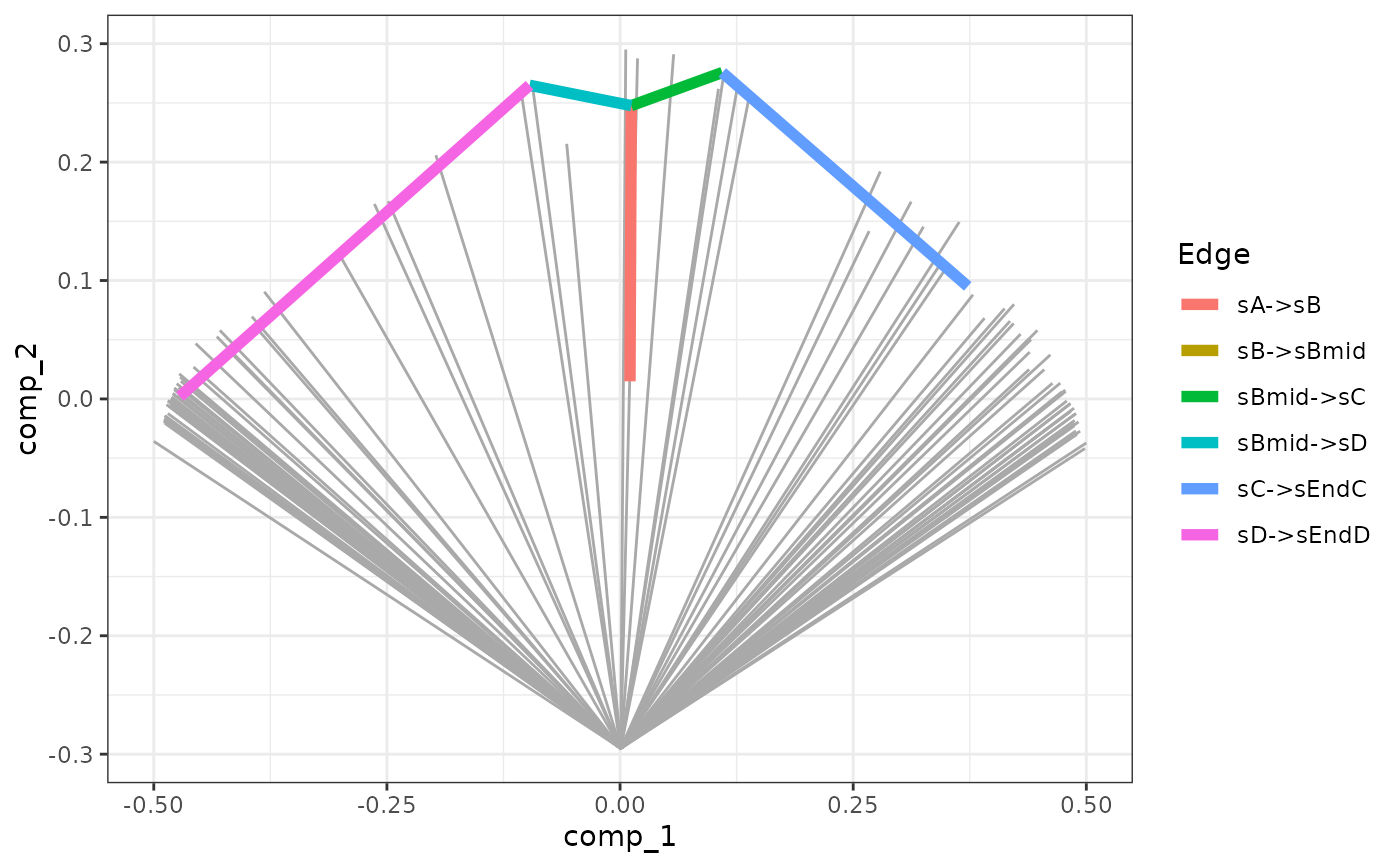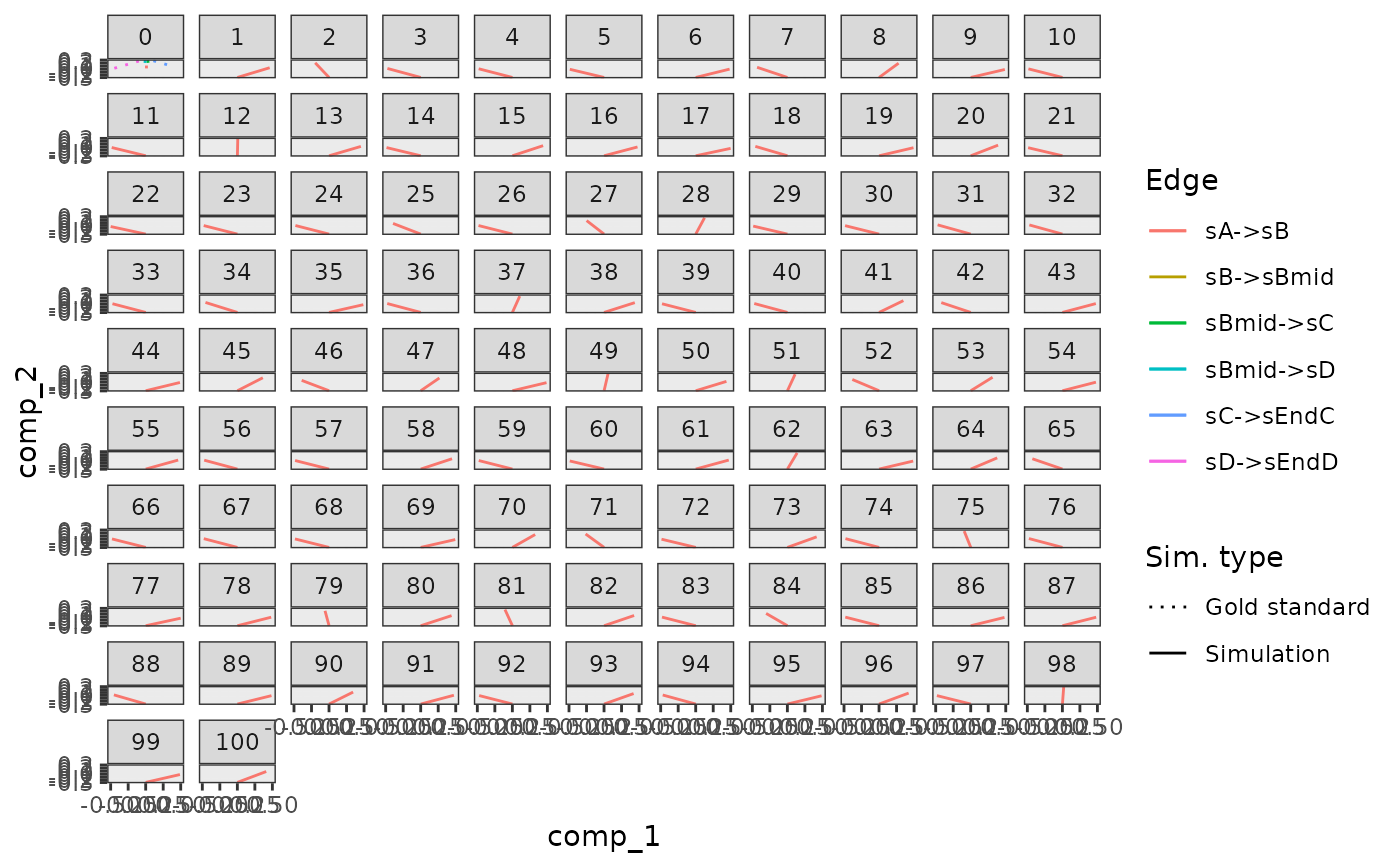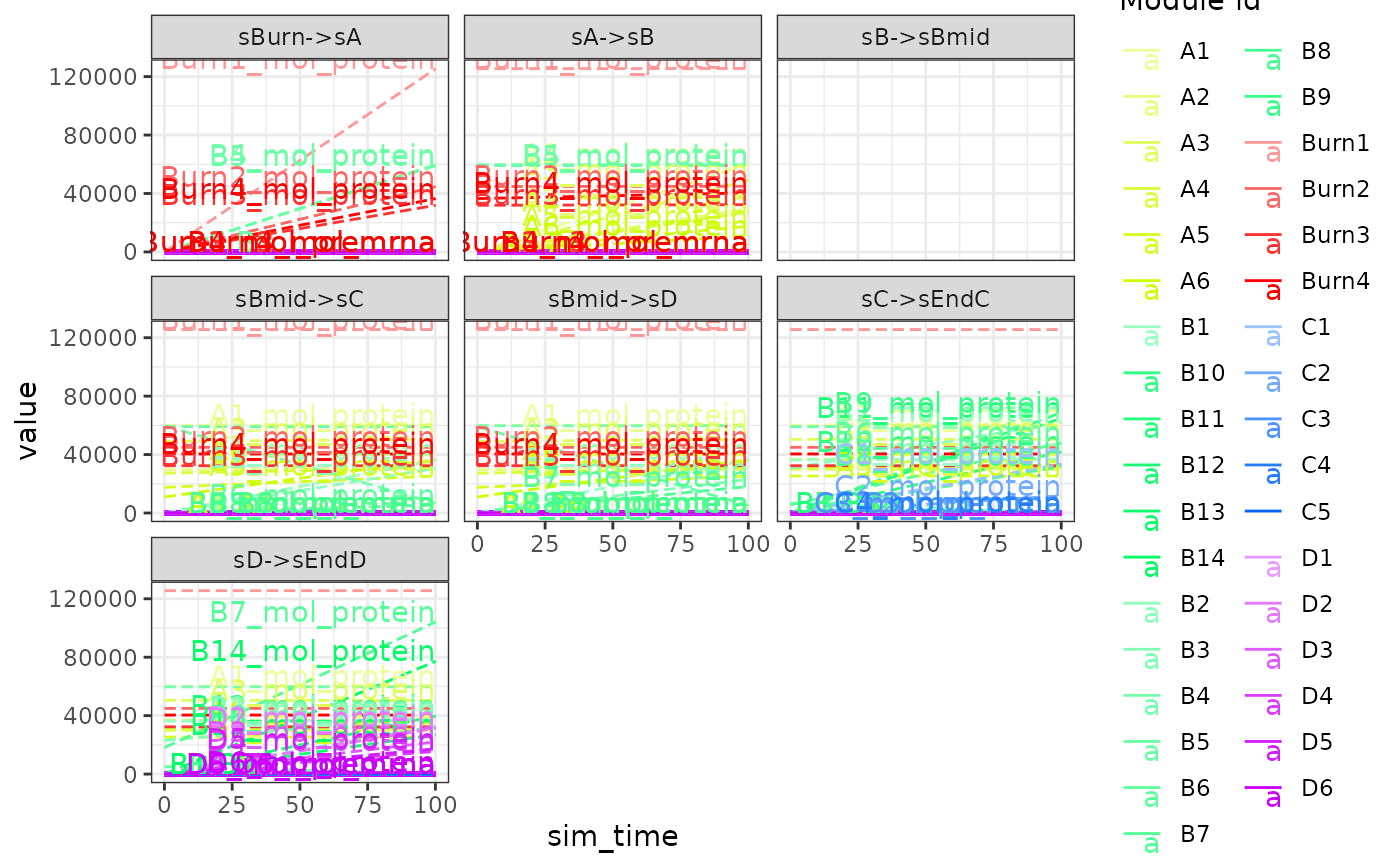generate_gold_standard() runs simulations in order to determine the gold standard of the simulations. gold_standard_default() is used to configure parameters pertaining this process.

generate_gold_standard(model)

gold_standard_default(
tau = 30/3600,
census_interval = 10/60,
simulate_targets = FALSE
)

## Arguments

model A dyngen intermediary model for which the kinetics of the feature network has been generated with generate_kinetics(). The time step of the ODE algorithm used to generate the gold standard. A granularity parameter of the gold standard time steps. Should be larger than or equal to tau. Also simulate the targets during the gold standard simulation

## Value

A dyngen model.

dyngen on how to run a complete dyngen simulation

## Examples

model <-
initialise_model(
backbone = backbone_bifurcating(),
gold_standard = gold_standard_default(tau = .01, census_interval = 1)
)

# \donttest{
data("example_model")
model <- example_model %>% generate_gold_standard()
#>
plot_gold_simulations(model)plot_gold_mappings(model)plot_gold_expression(model)
#> geom_path: Each group consists of only one observation. Do you need to adjust
#> the group aesthetic?# }